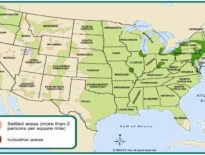The quadratic formula is a standard equation in algebra that can solve most quadratic equations. Review the equation and then practice by plugging in your own numbers and replacing the ‘A’, ‘B’ and ‘C’.

Once you have the quadratic formula mastered you can practice graphing quadratic functions.

Students take a broader look at computational and problem-solving skills while learning the language of algebra. Students translate word phrases and sentences into mathematical expressions; analyze geometric figures; solve problems involving percentages, ratios, and proportions; graph different kinds of equations and inequalities; calculate statistical measures and probabilities; apply the Pythagorean theorem; and explain strategies for solving real-world problems. Online lessons provide demonstrations of key concepts, as well as interactive problems with contextual feedback. A textbook supplements the online material. Students who take Pre-Algebra are expected to have mastered the skills and concepts presented in the K12 Fundamentals of Geometry and Algebra course (or equivalent).

Request a free catalog of K-12 Courses

Request the Scope & Sequence for a course

### Activity Center

#### Popular Articles